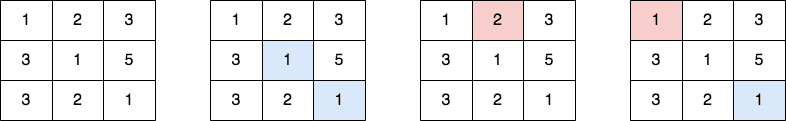# 2711. Difference of Number of Distinct Values on Diagonals

## Description

Given a 0-indexed 2D grid of size m x n, you should find the matrix answer of size m x n.

The value of each cell (r, c) of the matrix answer is calculated in the following way:

• Let topLeft[r][c] be the number of distinct values in the top-left diagonal of the cell (r, c) in the matrix grid.
• Let bottomRight[r][c] be the number of distinct values in the bottom-right diagonal of the cell (r, c) in the matrix grid.

Then answer[r][c] = |topLeft[r][c] - bottomRight[r][c]|.

Return the matrix answer.

A matrix diagonal is a diagonal line of cells starting from some cell in either the topmost row or leftmost column and going in the bottom-right direction until reaching the matrix's end.

A cell (r1, c1) belongs to the top-left diagonal of the cell (r, c), if both belong to the same diagonal and r1 < r. Similarly is defined bottom-right diagonal.

Example 1:Input: grid = [[1,2,3],[3,1,5],[3,2,1]]
Output: [[1,1,0],[1,0,1],[0,1,1]]
Explanation: The 1st diagram denotes the initial grid.
The 2nd diagram denotes a grid for cell (0,0), where blue-colored cells are cells on its bottom-right diagonal.
The 3rd diagram denotes a grid for cell (1,2), where red-colored cells are cells on its top-left diagonal.
The 4th diagram denotes a grid for cell (1,1), where blue-colored cells are cells on its bottom-right diagonal and red-colored cells are cells on its top-left diagonal.
- The cell (0,0) contains [1,1] on its bottom-right diagonal and [] on its top-left diagonal. The answer is |1 - 0| = 1.
- The cell (1,2) contains [] on its bottom-right diagonal and  on its top-left diagonal. The answer is |0 - 1| = 1.
- The cell (1,1) contains  on its bottom-right diagonal and  on its top-left diagonal. The answer is |1 - 1| = 0.
The answers of other cells are similarly calculated.


Example 2:

Input: grid = []
Output: []
Explanation: - The cell (0,0) contains [] on its bottom-right diagonal and [] on its top-left diagonal. The answer is |0 - 0| = 0.


Constraints:

• m == grid.length
• n == grid[i].length
• 1 <= m, n, grid[i][j] <= 50

## Solutions

• class Solution {
public int[][] differenceOfDistinctValues(int[][] grid) {
int m = grid.length, n = grid.length;
int[][] ans = new int[m][n];
for (int i = 0; i < m; ++i) {
for (int j = 0; j < n; ++j) {
int x = i, y = j;
Set<Integer> s = new HashSet<>();
while (x > 0 && y > 0) {
}
int tl = s.size();
x = i;
y = j;
s.clear();
while (x < m - 1 && y < n - 1) {
}
int br = s.size();
ans[i][j] = Math.abs(tl - br);
}
}
return ans;
}
}

• class Solution {
public:
vector<vector<int>> differenceOfDistinctValues(vector<vector<int>>& grid) {
int m = grid.size(), n = grid.size();
vector<vector<int>> ans(m, vector<int>(n));
for (int i = 0; i < m; ++i) {
for (int j = 0; j < n; ++j) {
int x = i, y = j;
unordered_set<int> s;
while (x > 0 && y > 0) {
s.insert(grid[--x][--y]);
}
int tl = s.size();
x = i;
y = j;
s.clear();
while (x < m - 1 && y < n - 1) {
s.insert(grid[++x][++y]);
}
int br = s.size();
ans[i][j] = abs(tl - br);
}
}
return ans;
}
};

• class Solution:
def differenceOfDistinctValues(self, grid: List[List[int]]) -> List[List[int]]:
m, n = len(grid), len(grid)
ans = [ * n for _ in range(m)]
for i in range(m):
for j in range(n):
x, y = i, j
s = set()
while x and y:
x, y = x - 1, y - 1
tl = len(s)
x, y = i, j
s = set()
while x + 1 < m and y + 1 < n:
x, y = x + 1, y + 1
br = len(s)
ans[i][j] = abs(tl - br)
return ans


• func differenceOfDistinctValues(grid [][]int) [][]int {
m, n := len(grid), len(grid)
ans := make([][]int, m)
for i := range grid {
ans[i] = make([]int, n)
for j := range grid[i] {
x, y := i, j
s := map[int]bool{}
for x > 0 && y > 0 {
x, y = x-1, y-1
s[grid[x][y]] = true
}
tl := len(s)
x, y = i, j
s = map[int]bool{}
for x+1 < m && y+1 < n {
x, y = x+1, y+1
s[grid[x][y]] = true
}
br := len(s)
ans[i][j] = abs(tl - br)
}
}
return ans
}

func abs(x int) int {
if x < 0 {
return -x
}
return x
}

• function differenceOfDistinctValues(grid: number[][]): number[][] {
const m = grid.length;
const n = grid.length;
const ans: number[][] = Array(m)
.fill(0)
.map(() => Array(n).fill(0));
for (let i = 0; i < m; ++i) {
for (let j = 0; j < n; ++j) {
let [x, y] = [i, j];
const s = new Set<number>();
while (x && y) {
}
const tl = s.size;
[x, y] = [i, j];
s.clear();
while (x + 1 < m && y + 1 < n) {